# Working with Missing Data in Pandas

## Working with Missing Data in Pandas

Missing Data can occur when no information is provided for one or more items or for a whole unit. Missing Data is a very big problem in a real-life scenarios. Missing Data can also refer to as `NA`(Not Available) values in pandas. In DataFrame sometimes many datasets simply arrive with missing data, either because it exists and was not collected or it never existed. For Example, Suppose different users being surveyed may choose not to share their income, some users may choose not to share the address in this way many datasets went missing.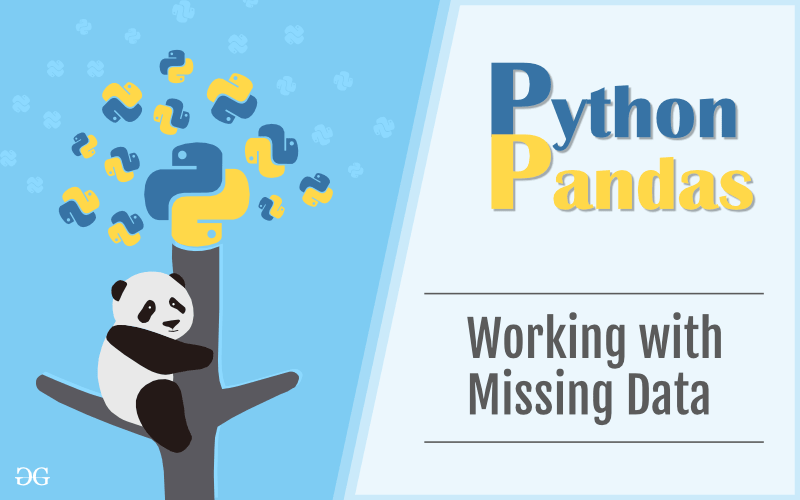In Pandas missing data is represented by two value:

• None: None is a Python singleton object that is often used for missing data in Python code.
• NaN : NaN (an acronym for Not a Number), is a special floating-point value recognized by all systems that use the standard IEEE floating-point representation

Pandas treat `None` and `NaN` as essentially interchangeable for indicating missing or null values. To facilitate this convention, there are several useful functions for detecting, removing, and replacing null values in Pandas DataFrame :

• isnull()
• notnull()
• dropna()
• fillna()
• replace()
• interpolate()

### Checking for missing values using `isnull()` and `notnull()`

In order to check missing values in Pandas DataFrame, we use a function `isnull()` and `notnull()`. Both function help in checking whether a value is `NaN` or not. These function can also be used in Pandas Series in order to find null values in a series.

#### Checking for missing values using `isnull()`

In order to check null values in Pandas DataFrame, we use `isnull()` function this function return dataframe of Boolean values which are True for NaN values.

Code #1:

 `# importing pandas as pd` `import` `pandas as pd` ` ` `# importing numpy as np` `import` `numpy as np` ` ` `# dictionary of lists` `dict` `=` `{``'First Score'``:[``100``, ``90``, np.nan, ``95``],` `        ``'Second Score'``: [``30``, ``45``, ``56``, np.nan],` `        ``'Third Score'``:[np.nan, ``40``, ``80``, ``98``]}` ` ` `# creating a dataframe from list` `df ``=` `pd.DataFrame(``dict``)` ` ` `# using isnull() function  ` `df.isnull()`

Output: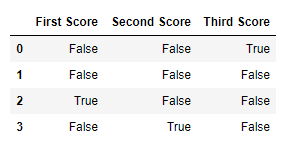Code #2:

 `# importing pandas package ` `import` `pandas as pd ` `   ` `# making data frame from csv file ` `data ``=` `pd.read_csv(``"employees.csv"``) ` `   ` `# creating bool series True for NaN values ` `bool_series ``=` `pd.isnull(data[``"Gender"``]) ` `   ` `# filtering data ` `# displaying data only with Gender = NaN ` `data[bool_series] `

Output:
As shown in the output image, only the rows having `Gender = NULL` are displayed.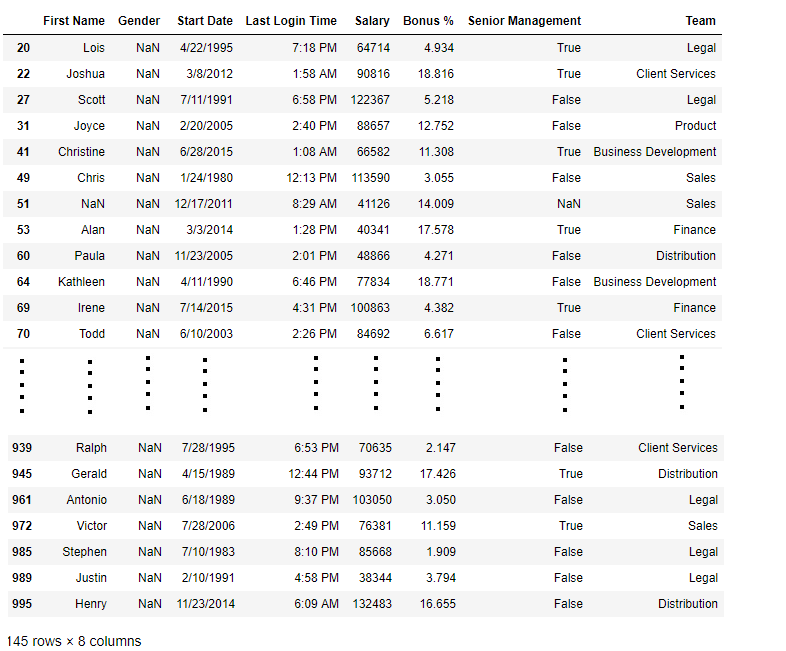#### Checking for missing values using `notnull()`

In order to check null values in Pandas Dataframe, we use notnull() function this function return dataframe of Boolean values which are False for NaN values.

Code #3:

 `# importing pandas as pd` `import` `pandas as pd` ` ` `# importing numpy as np` `import` `numpy as np` ` ` `# dictionary of lists` `dict` `=` `{``'First Score'``:[``100``, ``90``, np.nan, ``95``],` `        ``'Second Score'``: [``30``, ``45``, ``56``, np.nan],` `        ``'Third Score'``:[np.nan, ``40``, ``80``, ``98``]}` ` ` `# creating a dataframe using dictionary` `df ``=` `pd.DataFrame(``dict``)` ` ` `# using notnull() function ` `df.notnull()`

Output: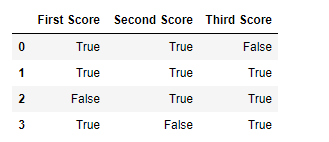Code #4:

 `# importing pandas package ` `import` `pandas as pd ` `   ` `# making data frame from csv file ` `data ``=` `pd.read_csv(``"employees.csv"``) ` `   ` `# creating bool series True for NaN values ` `bool_series ``=` `pd.notnull(data[``"Gender"``]) ` `   ` `# filtering data ` `# displayind data only with Gender = Not NaN ` `data[bool_series] `

Output:
As shown in the output image, only the rows having `Gender = NOT NULL` are displayed.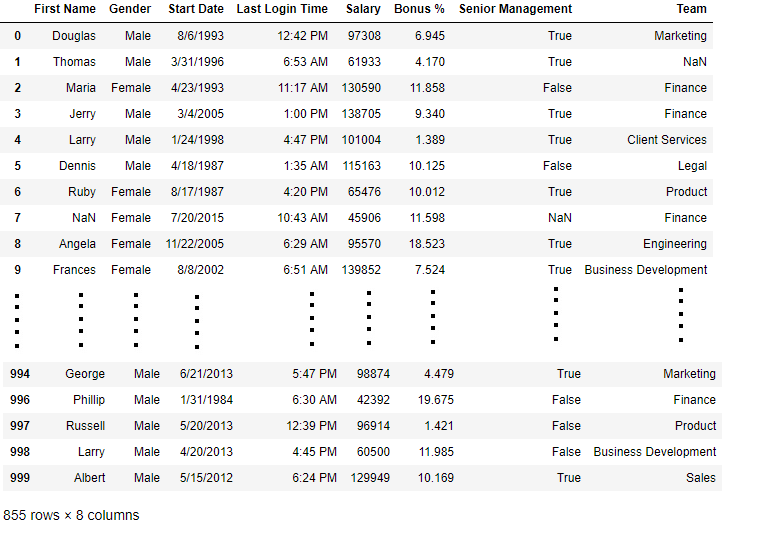### Filling missing values using `fillna()`, `replace()` and `interpolate()`

In order to fill null values in a datasets, we use `fillna()``replace()` and `interpolate()` function these function replace NaN values with some value of their own. All these function help in filling a null values in datasets of a DataFrame. Interpolate() function is basically used to fill `NA` values in the dataframe but it uses various interpolation technique to fill the missing values rather than hard-coding the value.

Code #1: Filling null values with a single value

 `# importing pandas as pd` `import` `pandas as pd` ` ` `# importing numpy as np` `import` `numpy as np` ` ` `# dictionary of lists` `dict` `=` `{``'First Score'``:[``100``, ``90``, np.nan, ``95``],` `        ``'Second Score'``: [``30``, ``45``, ``56``, np.nan],` `        ``'Third Score'``:[np.nan, ``40``, ``80``, ``98``]}` ` ` `# creating a dataframe from dictionary` `df ``=` `pd.DataFrame(``dict``)` ` ` `# filling missing value using fillna()  ` `df.fillna(``0``)`

Output: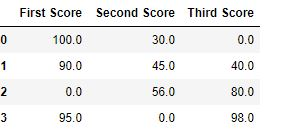Code #2: Filling null values with the previous ones

 `# importing pandas as pd` `import` `pandas as pd` ` ` `# importing numpy as np` `import` `numpy as np` ` ` `# dictionary of lists` `dict` `=` `{``'First Score'``:[``100``, ``90``, np.nan, ``95``],` `        ``'Second Score'``: [``30``, ``45``, ``56``, np.nan],` `        ``'Third Score'``:[np.nan, ``40``, ``80``, ``98``]}` ` ` `# creating a dataframe from dictionary` `df ``=` `pd.DataFrame(``dict``)` ` ` `# filling a missing value with` `# previous ones  ` `df.fillna(method ``=``'pad'``)`

Output: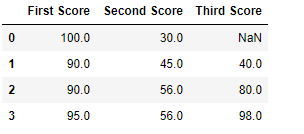Code #3: Filling null value with the next ones

 `# importing pandas as pd` `import` `pandas as pd` ` ` `# importing numpy as np` `import` `numpy as np` ` ` `# dictionary of lists` `dict` `=` `{``'First Score'``:[``100``, ``90``, np.nan, ``95``],` `        ``'Second Score'``: [``30``, ``45``, ``56``, np.nan],` `        ``'Third Score'``:[np.nan, ``40``, ``80``, ``98``]}` ` ` `# creating a dataframe from dictionary` `df ``=` `pd.DataFrame(``dict``)` ` ` `# filling  null value using fillna() function  ` `df.fillna(method ``=``'bfill'``)`

Output: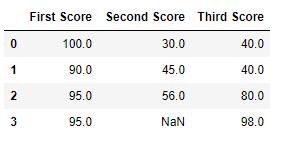Code #4: Filling null values in CSV File

 `# importing pandas package ` `import` `pandas as pd ` `   ` `# making data frame from csv file ` `data ``=` `pd.read_csv(``"employees.csv"``)` ` ` `# Printing the first 10 to 24 rows of` `# the data frame for visualization   ` `data[``10``:``25``]`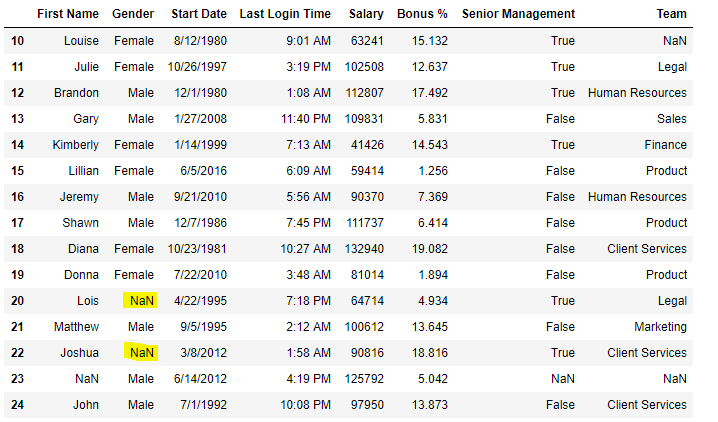Now we are going to fill all the null values in Gender column with “No Gender”

 `# importing pandas package ` `import` `pandas as pd ` `   ` `# making data frame from csv file ` `data ``=` `pd.read_csv(``"employees.csv"``) ` ` ` `# filling a null values using fillna() ` `data[``"Gender"``].fillna(``"No Gender"``, inplace ``=` `True``) ` ` ` `data`

Output: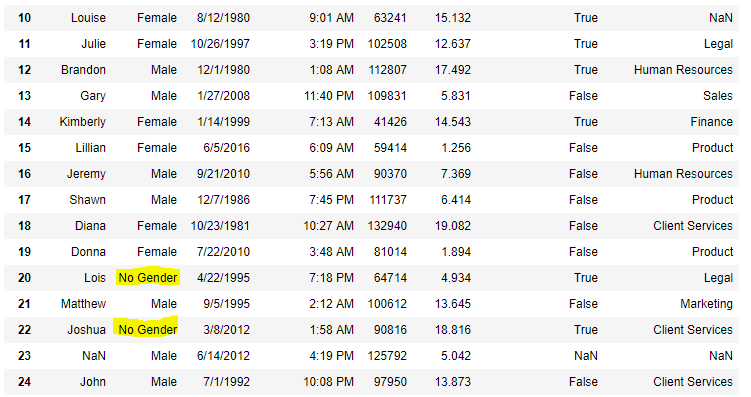Code #5: Filling a null values using replace() method

 `# importing pandas package ` `import` `pandas as pd ` `   ` `# making data frame from csv file ` `data ``=` `pd.read_csv(``"employees.csv"``)` ` ` `# Printing the first 10 to 24 rows of` `# the data frame for visualization   ` `data[``10``:``25``]`

Output: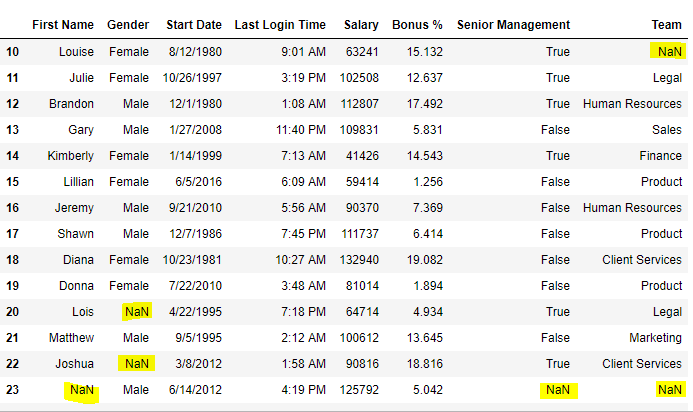Now we are going to replace the all Nan value in the data frame with -99 value.

 `# importing pandas package ` `import` `pandas as pd ` `   ` `# making data frame from csv file ` `data ``=` `pd.read_csv(``"employees.csv"``) ` `   ` `# will replace  Nan value in dataframe with value -99  ` `data.replace(to_replace ``=` `np.nan, value ``=` `-``99``) `

Output: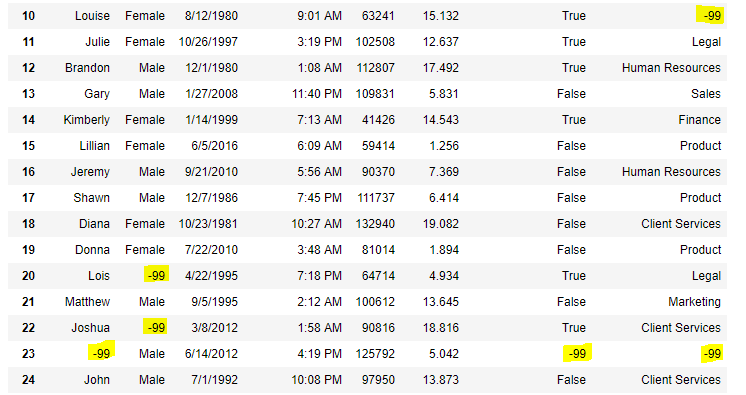Code #6: Using interpolate() function to fill the missing values using linear method.

 `# importing pandas as pd ` `import` `pandas as pd ` `   ` `# Creating the dataframe  ` `df ``=` `pd.DataFrame({``"A"``:[``12``, ``4``, ``5``, ``None``, ``1``], ` `                   ``"B"``:[``None``, ``2``, ``54``, ``3``, ``None``], ` `                   ``"C"``:[``20``, ``16``, ``None``, ``3``, ``8``], ` `                   ``"D"``:[``14``, ``3``, ``None``, ``None``, ``6``]}) ` `   ` `# Print the dataframe ` `df `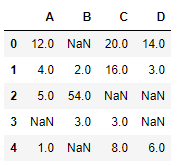Let’s interpolate the missing values using Linear method. Note that Linear method ignore the index and treat the values as equally spaced.

 `# to interpolate the missing values ` `df.interpolate(method ``=``'linear'``, limit_direction ``=``'forward'``)`

Output: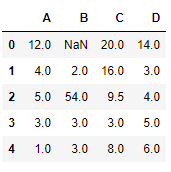As we can see the output, values in the first row could not get filled as the direction of filling of values is forward and there is no previous value which could have been used in interpolation.

### Dropping missing values using `dropna()`

In order to drop a null values from a dataframe, we used `dropna()` function this function drop Rows/Columns of datasets with Null values in different ways.

Code #1: Dropping rows with at least 1 null value.

 `# importing pandas as pd` `import` `pandas as pd` ` ` `# importing numpy as np` `import` `numpy as np` ` ` `# dictionary of lists` `dict` `=` `{``'First Score'``:[``100``, ``90``, np.nan, ``95``],` `        ``'Second Score'``: [``30``, np.nan, ``45``, ``56``],` `        ``'Third Score'``:[``52``, ``40``, ``80``, ``98``],` `        ``'Fourth Score'``:[np.nan, np.nan, np.nan, ``65``]}` ` ` `# creating a dataframe from dictionary` `df ``=` `pd.DataFrame(``dict``)` `   ` `df`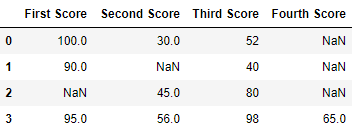Now we drop rows with at least one Nan value (Null value)

 `# importing pandas as pd` `import` `pandas as pd` ` ` `# importing numpy as np` `import` `numpy as np` ` ` `# dictionary of lists` `dict` `=` `{``'First Score'``:[``100``, ``90``, np.nan, ``95``],` `        ``'Second Score'``: [``30``, np.nan, ``45``, ``56``],` `        ``'Third Score'``:[``52``, ``40``, ``80``, ``98``],` `        ``'Fourth Score'``:[np.nan, np.nan, np.nan, ``65``]}` ` ` `# creating a dataframe from dictionary` `df ``=` `pd.DataFrame(``dict``)` ` ` `# using dropna() function  ` `df.dropna()`

Output: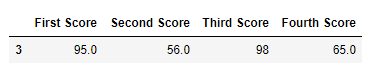Code #2: Dropping rows if all values in that row are missing.

 `# importing pandas as pd` `import` `pandas as pd` ` ` `# importing numpy as np` `import` `numpy as np` ` ` `# dictionary of lists` `dict` `=` `{``'First Score'``:[``100``, np.nan, np.nan, ``95``],` `        ``'Second Score'``: [``30``, np.nan, ``45``, ``56``],` `        ``'Third Score'``:[``52``, np.nan, ``80``, ``98``],` `        ``'Fourth Score'``:[np.nan, np.nan, np.nan, ``65``]}` ` ` `# creating a dataframe from dictionary` `df ``=` `pd.DataFrame(``dict``)` `   ` `df`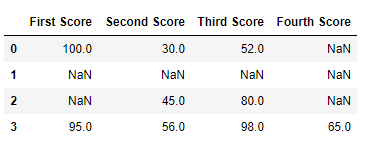Now we drop a rows whose all data is missing or contain null values(NaN)

 `# importing pandas as pd` `import` `pandas as pd` ` ` `# importing numpy as np` `import` `numpy as np` ` ` `# dictionary of lists` `dict` `=` `{``'First Score'``:[``100``, np.nan, np.nan, ``95``],` `        ``'Second Score'``: [``30``, np.nan, ``45``, ``56``],` `        ``'Third Score'``:[``52``, np.nan, ``80``, ``98``],` `        ``'Fourth Score'``:[np.nan, np.nan, np.nan, ``65``]}` ` ` `df ``=` `pd.DataFrame(``dict``)` ` ` `# using dropna() function    ` `df.dropna(how ``=` `'all'``)`

Output: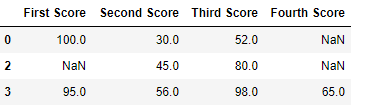Code #3: Dropping columns with at least 1 null value.

 `# importing pandas as pd` `import` `pandas as pd` `  ` `# importing numpy as np` `import` `numpy as np` `  ` `# dictionary of lists` `dict` `=` `{``'First Score'``:[``100``, np.nan, np.nan, ``95``],` `        ``'Second Score'``: [``30``, np.nan, ``45``, ``56``],` `        ``'Third Score'``:[``52``, np.nan, ``80``, ``98``],` `        ``'Fourth Score'``:[``60``, ``67``, ``68``, ``65``]}` ` ` `# creating a dataframe from dictionary ` `df ``=` `pd.DataFrame(``dict``)` `    ` `df`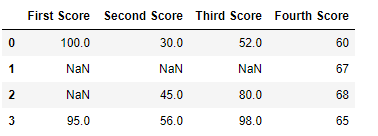Now we drop a columns which have at least 1 missing values

 `# importing pandas as pd` `import` `pandas as pd` `  ` `# importing numpy as np` `import` `numpy as np` `  ` `# dictionary of lists` `dict` `=` `{``'First Score'``:[``100``, np.nan, np.nan, ``95``],` `        ``'Second Score'``: [``30``, np.nan, ``45``, ``56``],` `        ``'Third Score'``:[``52``, np.nan, ``80``, ``98``],` `        ``'Fourth Score'``:[``60``, ``67``, ``68``, ``65``]}` ` ` `# creating a dataframe from dictionary  ` `df ``=` `pd.DataFrame(``dict``)` ` ` `# using dropna() function     ` `df.dropna(axis ``=` `1``)`

Output :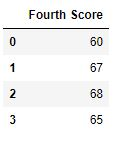Code #4: Dropping Rows with at least 1 null value in CSV file

 `# importing pandas module ` `import` `pandas as pd ` `   ` `# making data frame from csv file ` `data ``=` `pd.read_csv(``"employees.csv"``) ` `   ` `# making new data frame with dropped NA values ` `new_data ``=` `data.dropna(axis ``=` `0``, how ``=``'any'``) ` `   ` `new_data`

Output: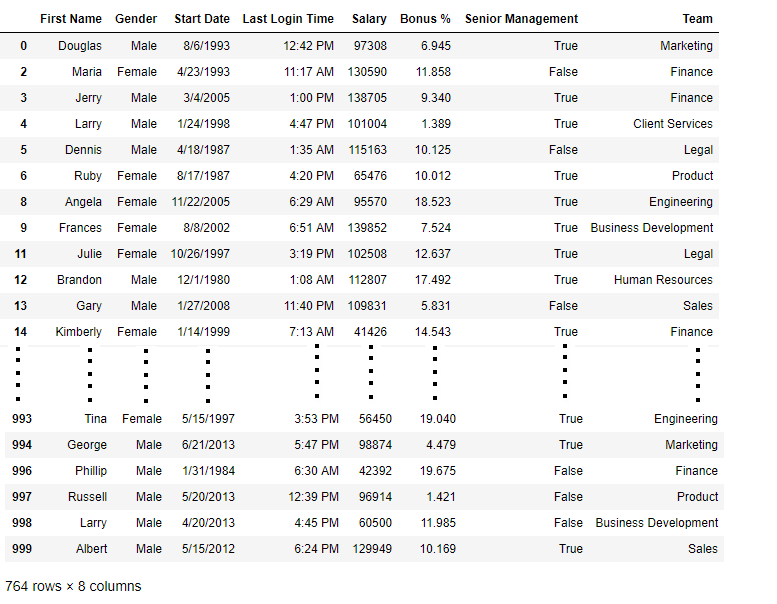Now we compare sizes of data frames so that we can come to know how many rows had at least 1 Null value

 `print``(``"Old data frame length:"``, ``len``(data))` `print``(``"New data frame length:"``, ``len``(new_data)) ` `print``(``"Number of rows with at least 1 NA value: "``, (``len``(data)``-``len``(new_data)))`

Output :

```Old data frame length: 1000
New data frame length: 764
Number of rows with at least 1 NA value:  236
```

Since the difference is 236, there were 236 rows which had at least 1 Null value in any column.

Last Updated on October 24, 2021 by admin

## Density Plots with Pandas in PythonDensity Plots with Pandas in Python

Density Plots with Pandas in Python Density Plot is a type of data visualization tool.

## Pandas Series.str.startswith()Pandas Series.str.startswith()

Python | Pandas Series.str.startswith() Python is a great language for doing data analysis, primarily because

## Check if dataframe contains infinity in Python – PandasCheck if dataframe contains infinity in Python – Pandas

When working with data in Python using Pandas, it is important to ensure that the

## Python – Pandas dataframe.append()Python – Pandas dataframe.append()

Python – Pandas dataframe.append() Pandas dataframe.append() function is used to append rows of other dataframe

## Heap queue (or heapq) in PythonHeap queue (or heapq) in Python

Heap queue (or heapq) in Python Heap data structure is mainly used to represent a

## Count NaN or missing values in Pandas DataFrameCount NaN or missing values in Pandas DataFrame

Count NaN or missing values in Pandas DataFrame   In this article, we will see

## Working with excel files using PandasWorking with excel files using Pandas

Working with excel files using Pandas Excel sheet is one of the most ubiquitous forms

## Detect and Remove the Outliers using PythonDetect and Remove the Outliers using Python

Detect and Remove the Outliers using Python An Outlier is a data-item/object that deviates significantly